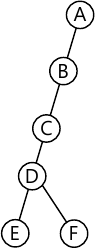MORE IN Data Structures and Applications
VTU Computer Science (Semester 3)
Data Structures and Applications
June 2015
Total marks: --
Total time: --
INSTRUCTIONS
(1) Assume appropriate data and state your reasons
(2) Marks are given to the right of every question
(3) Draw neat diagrams wherever necessary

1 (a) What is an algorithm? Briefly explain the criteria that an algorithm must satisfy.
6 M
1 (b) Write an recursive function to sum a list of numbers and also show the total step counts for the function.
7 M
1 (c) Define three asymptotic notations and give the asymptotic representation of function 10n+5 in all the three notations.
7 M

2 (a) What is a structure? Give three different ways of defining structure with example to each.
7 M
2 (b) What is the degree of the polynomial? Consider the two polynomials A(x)-x1000+1 and B(x)=10x3+3x2+1. Show diagrammatically how these two polynomials can be represented in an array.
5 M
2 (c) For the given sparse matrix and its transpose, give the triplet using one dimensional array, A is the given sparse matrix, B will be its transpose. $A = \begin{bmatrix} 15 &0 &0 &22 &0 &-15 \\0 &11 &3 &0 &0 &0 \\0 &0 &0 &-6 &0 &0 \\0 &0 &0 &0 &0 &0 \\ -91 &0 &0 &0 &0 &0 \\0 &0 &28 &0 &0 &0 \end{bmatrix}$
8 M

3 (a) Convert the following infix expression into postfix expression using stack: $i) \ a* (b+c)*b \\ ii) \ \dfrac {(a+b)* d+e}{(f+a*d)+c}$
8 M
3 (b) Write a C function to evaluate a postfix expression and apply the same to evaluate AB+CDE -*/, A=5, B=6, C=4, D=3, E=7.
12 M

4 (a) Write a C function to insert a node at front and rear end in a circular linked list.
10 M
4 (b) (i) Write a C function to reverse the given singly linked list.
5 M
4 (b) (ii) Write a C function to concatenate two singly linked list.
5 M

5 (a) What is a binary tree? Show the array representation and linked representation for the following binary tree.5 M
5 (b) Write an expression tree for the expression A/B*C*D+E. Give the C function for inorder, preorder, postorder traversals and apply the traversal methods to the expression tree and give the result of traversals.
10 M
5 (c) What is a max heap? Construct the max heap for 7, 8, 3, 6, 9, 4, 10, 5.
5 M

6 (a) What is a binary search tree? Draw the binary tree for input:
14, 15, 4, 9, 7, 18, 3, 5, 16, 4, 20, 17, 9.
Give recursive search function to search an element in that tree.
10 M
6 (b) Construct the binary tree from the given traversals:
Preorder: A B D G C E H I F
Inorder: D G B A H E I C F
5 M
6 (c) What is a winner tree? Explain with suitable example a winner tree for k=8.
5 M

7 (a) What is Fibonacci Heap? Give example. Give the steps for election of node and decrease key of specified node in F-heap.
10 M
7 (b) Write short note on: i) Binontial heaps
ii) Leftist trees.
10 M

8 (a) Starting with an empty AVL tree perform following sequence of insertion, MARCH, MAX, NOVEMBER, AUGUST, APRIL, JANUARY, DECEMBER, JULY. Draw the AVL tree following each insertion and state rotation type if any for insertion operation.
10 M
8 (b) Explain the red-black tree with example.
6 M
8 (c) Let h be the height of a red-black tree, let n be the number of internal nodes in the tree and r be the rank of the root then, prove that
i) h ≤ 2r
ii) n ≥ 2r-1.
4 M

More question papers from Data Structures and Applications